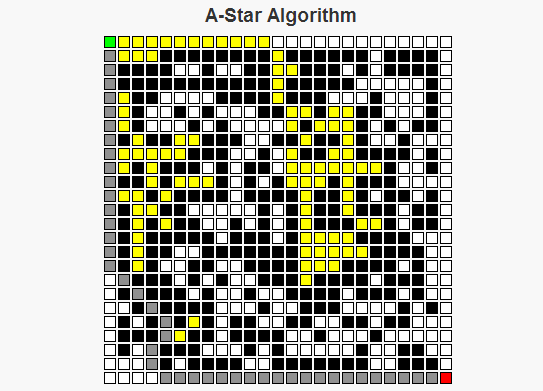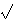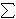# The A* AlgorithmAs a child I remember traveling on road trips, sitting in the back of a car trying to do my best to keep myself busy for what would occupy the next six to ten hours of my life. One of the things I grew to like were the simple maze books that were sold on the magazine racks at some of the gas stations where we’d stop for food. There are two basic strategies I employed for solving these mazes: For simpler mazes, I could generally just take a look at the overall maze structure, decide upon a path through the maze, then write a path without any mistakes. For more complex mazes though, I would generally begin a route that looks the most promising. If that route reaches a point where I can see that it will be impossible to finish, then I’d go back to where the decision was made, exclude the route I had just tried, and select the “most promising” remaining route. I’d continue this process until I had completed the maze, or until it became simple enough for me to solve the maze using only my memory.

The A* Algorithm works in a similar manner to the second approach I just described. We begin at a starting point, and consider where to move next from that starting point. The set of possible options for this next move is determined by the neighbor function for a given cell. For each neighbor the algorithm estimates the length of the route through that cell by calculating the sum of two values, f(n) = g(n) + h(n), where

g(n) is the (known) cost to travel from the starting point to the cell n.
h(n) is the (approximate) cost to travel from the cell n to the final cell.

The sum g(n) + h(n) allows us to approximate the total cost of a route through the cell n.

The A* algorithm begins at the starting position of the maze. There are two sets we will be considering throughout the process of determining the optimal route, called the closedSet and the openSet. The elements of closedSet are the nodes whose total distance from the starting position has been calculated, whereas the elements of openSet represents nodes whose total distance is still under consideration. There is also a map called prev which is used to reconstruct the path. Below is how the algorithm operates:

A* Algorithm Pseudocode
closedSet is the empty set
openSet = {start}
prev is the empty set

While there are still elements in openSet,
Find the element c* in openSet with the lowest value f(c).
If c* is the target position
Reconstruct the path.
Else If c* is not the target position,
Remove c* from openSet
Add c* to closedSet
For each neighbor n of c* that is not in closedSet,
Calculate a temporary g value, temp_g(n) = g(c*) + dist(c*, n).
If n is not in openSet, or if n is in openSet and temp_g(n) < g(n),                     Set prev(n) = c*                     Set g(n) = temp_g(n)                     Set f(n) = g(n) + h(n).                     if n is not in openSet                          add n to openSet.      End If End While End Algorithm An important question becomes what makes a good heuristic function to approximate the distance to the goal. This can lead to an in depth discussion based on the word "good", but the necessary condition for any heuristic is that it NEVER over-estimates the cost of the path from the cell to the goal. Some examples of possible heuristics for mazes are the Euclidean Distance (the square root of the sum of the squares of the horizontal and vertical differences in distances, i.e. dE(x, y) =(i(xi – yi)2) and TaxiCab Distance (the sum of the differences in the horizontal and vertical dimensions, i.e. dT(x, y) =i|xi – yi|). Both of these are feasible metrics for heuristics on a maze. Other herusitics, like h(n) = 0 for all n are possible, but doing this would make the algorithm treat all cells equally and ignore the heuristic part of the A* algorithm, turning it into Dijkstra’s algorithm.

I’ve written a script that generates random mazes and uses the A* algorithm to find the optimal path through this maze. For this script, I used the taxicab distance heuristic.

Check it out and let me know what you think.